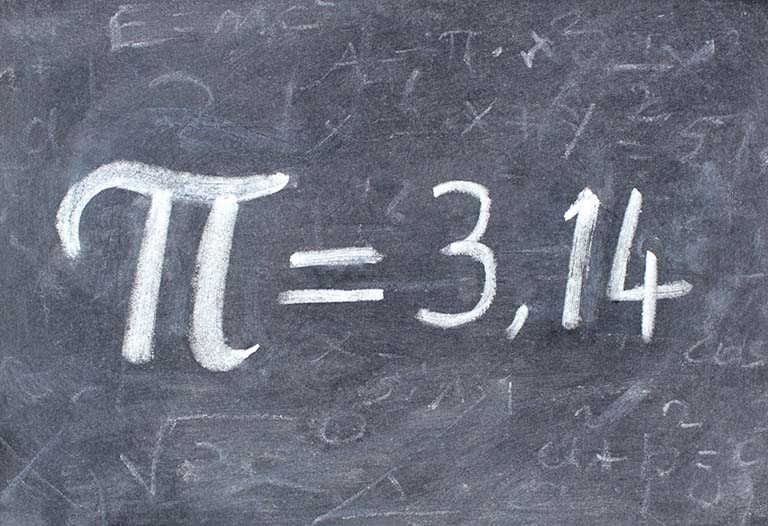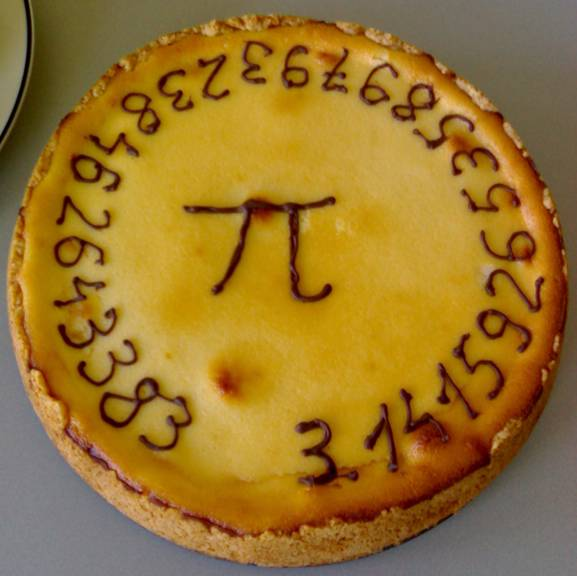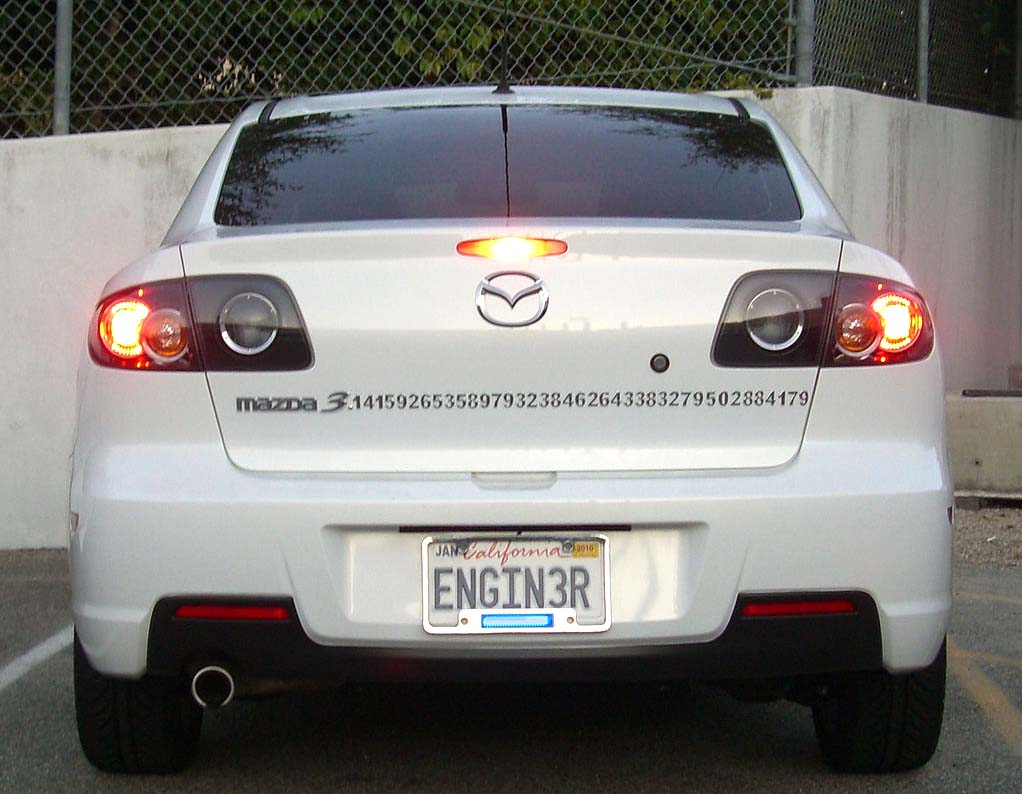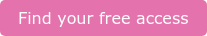Pi. It's 3.14159...ish?  Or is it 3.14159265359 and so on and so forth?

As tomorrow is Pi Day, an annual celebration of the mathematical constant π (Pi), we wanted to share our thoughts on the significance of the number and what you can do to celebrate.

We know that Pi is irrational, that it almost certainly never settles into a pattern. We hear all the time about computers trying to calculate the hundred-trillionth digit of Pi. Why? It's possible that something really interesting happens with Pi when you get enough digits, but it seems unlikely.### How much difference does it make?

We know that the circumference of a circle is equal to 2.π.r. So, if we know that the earth's radius is 6,371 (although that varies as the earth is not perfectly round), we can work out the earth's circumference c.c = 2.π.6371, so c = 12742.π. If we take Pi as 3.14, we find c to be 40009.88 km.

If we take Pi as 3.14159265358979323846264338327950288420 (rounded to 39 digits), we find c to be 40030 km (rounded to the nearest kilometre).However, if we take pi as 3.14159, we also find c to be 40030 km.

Clearly we don't need that many digits of Pi. In fact, we (roughly) only need as many digits of Pi as we need significant figures in our answer. Anything else is - for this particular purpose - needless precision. In fact if we use my favourite Pi approximation, the fracion 227 , we'd get surprisingly close at 40046 km.Image: Pi Pie at Delft University    Source: Wikipedia

### So, how many digits of Pi could we ever really need?

Suppose you needed to work out the circumference of the observable universe to within the width of a hydrogen atom? The answer is surprisingly small: 39. You need 39 digits (as shown above) to compare something so impossibly vast to something too small to be seen without an electron microscope.

So this Pi day, enjoy a slice of your favourite (I strongly recommend fresh peach pie), and try not to over do it on digits or servings. And if you drive a Mazda 3, you might want to do this.Image: A Pi-loving Mazda 3 owner extended the car's logo    Source: Wikipedia

(Note: The circumference of the earth is much closer to 40,075 km. The earth's circumference and radius both vary a little as the planet is a shape called an "oblate spheroid".)

If you have a maths problem you need help with, get in touch with one of our expert maths Specialists who are there to help at the click of a button.